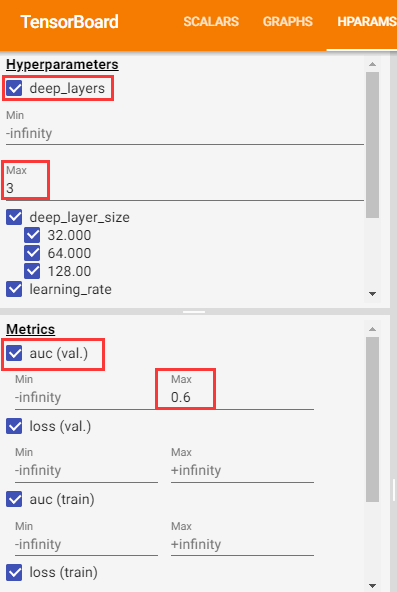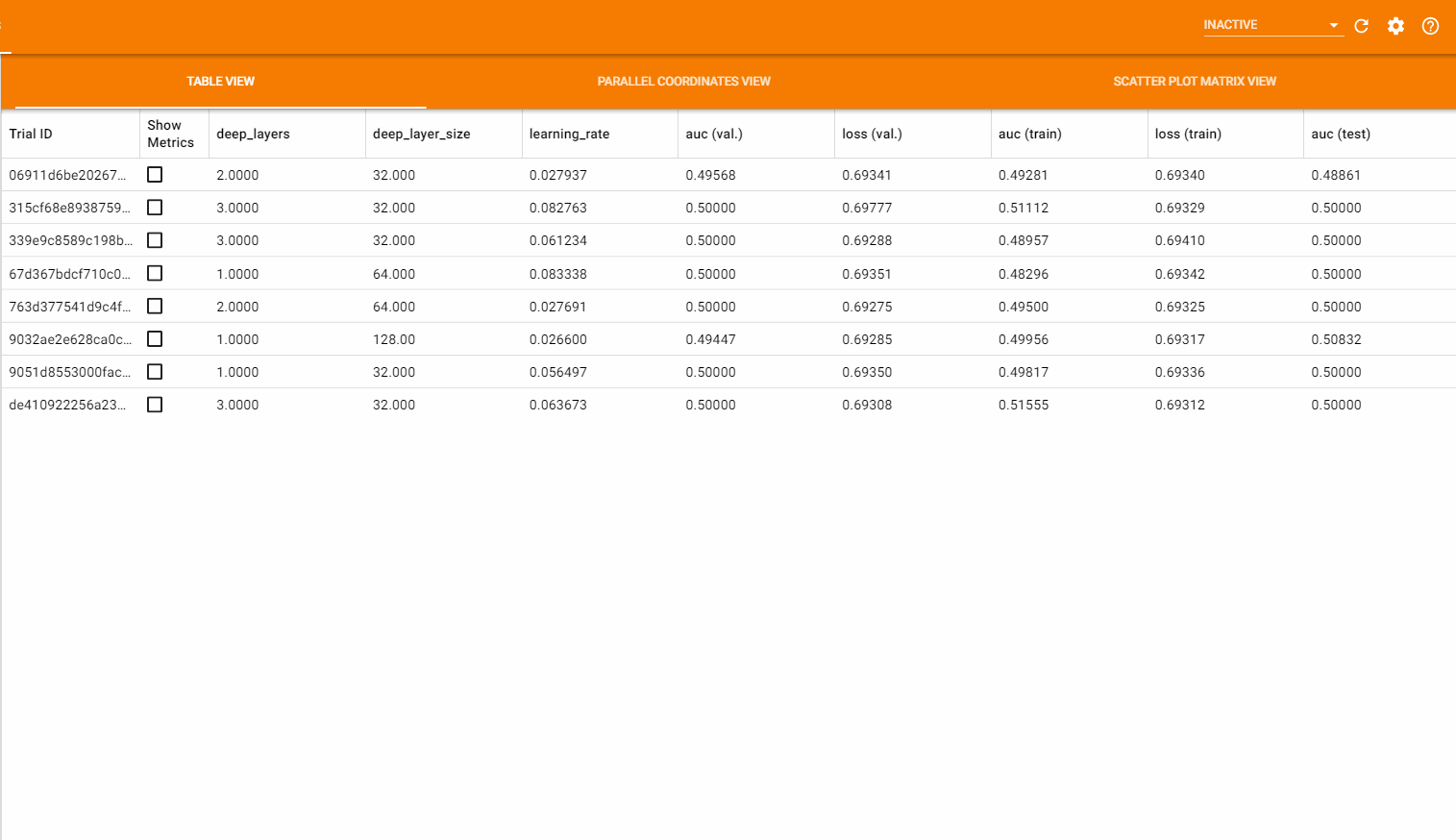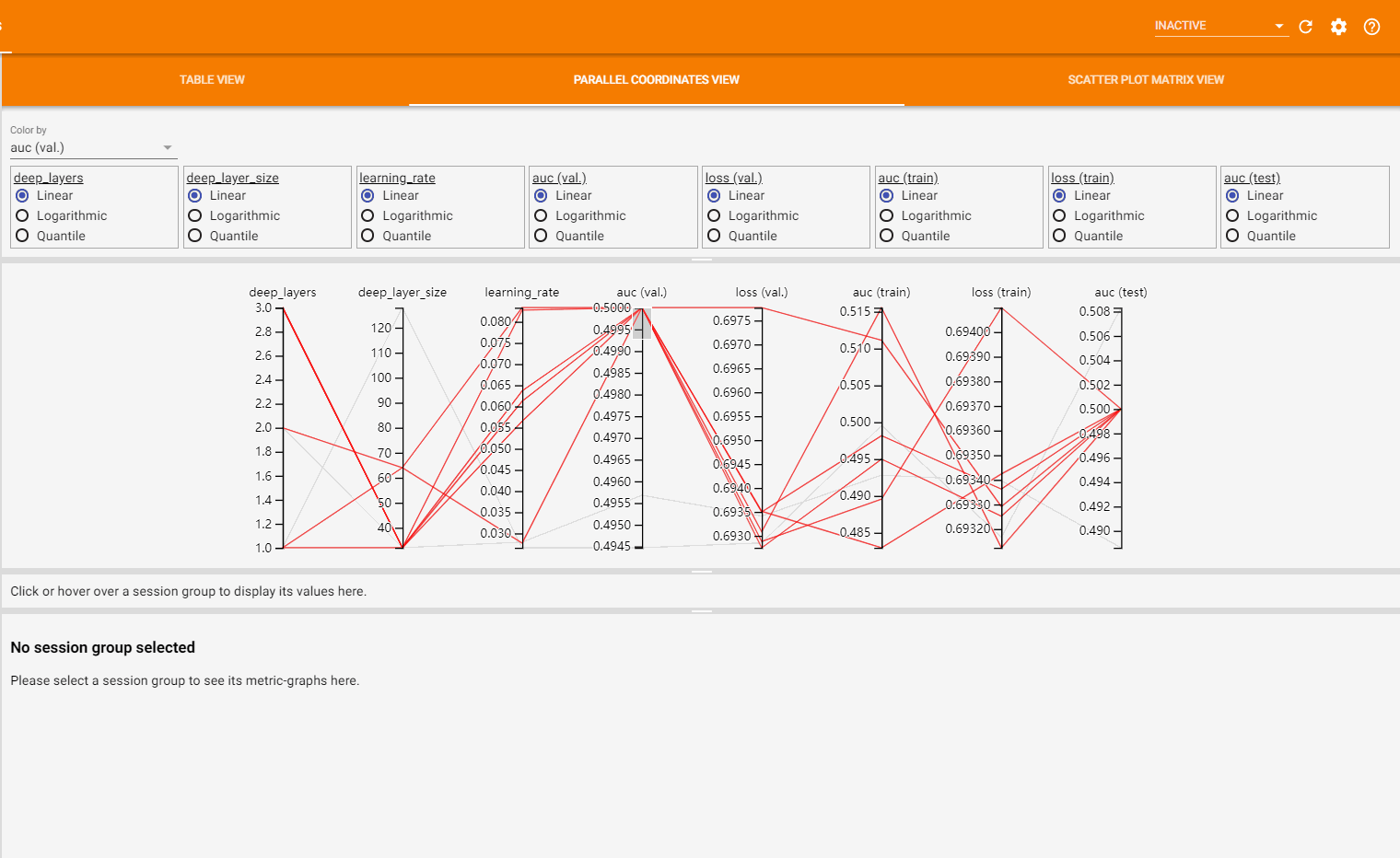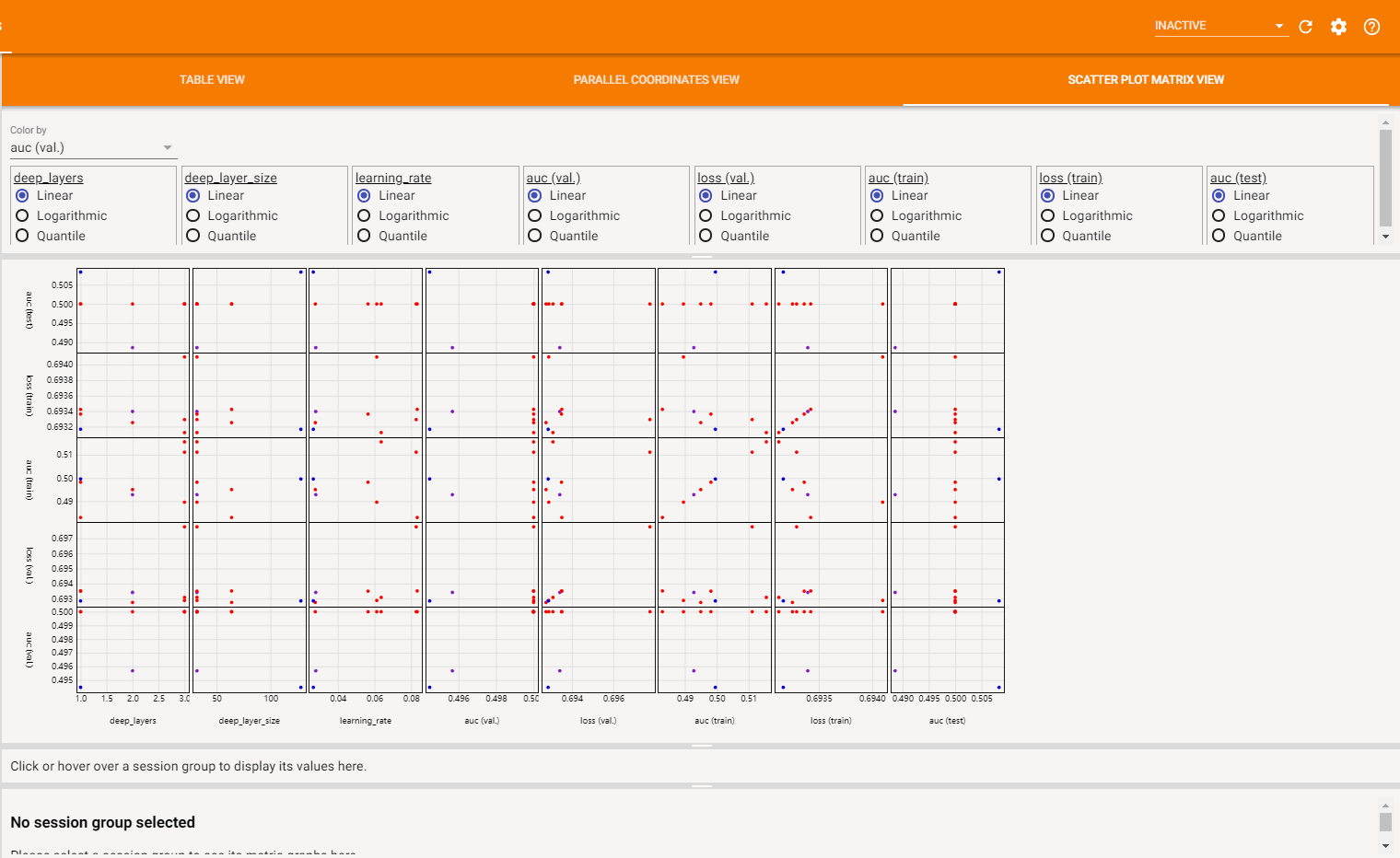TensorFlow 篇 | TensorFlow 2.x 基于 HParams 的超参数调优

TensorFlow 篇 | TensorFlow 2.x 基于 HParams 的超参数调优

2021-08-16 9:31:5869超参数调优策略

网格搜索

1. 网格搜索是指在所有候选的超参数取值中，尝试所有的超参数组合，并选取其中使模型效果达到最优的超参数组合作为最终解。

2. 比如模型有 2 个超参数，每个超参数有 3 个候选值，那么所有的超参数组合就有 3*3=9 种，网格搜索会尝试所有 9 组超参数并从中选择最佳组合。

3. 网格搜索的缺点是，模型训练评估的次数会随超参数数量以及超参数候选值数量的增加而呈指数级增长，从而产生很高的算力和时间成本，因此它并不适用于超参数的数量及候选值数量较多的情况。

随机搜索

1. 随机搜索是指每次选取随机的超参数组合进行尝试，它可以手动设置尝试的次数，避免遍历整个超参数空间，从而可以减少尝试的成本。

2. 相比网格搜索，随机搜索能够更高效地进行超参数调优，但是随机搜索并不能保证一定能选取到最优的超参数取值，具有不确定性。

HParams 超参数调优步骤

TensorFlow 提供了 HParams 插件来辅助我们进行超参数调优， HParams 插件支持网格搜索和随机搜索两种策略。下面以 Keras 模型训练为例，介绍使用 HParams 进行超参数调优的步骤。

定义超参数

1. 使用 HParam 类初始化定义所有的超参数，并指定超参数的取值域 (Domain)。 Domain 有三种类型，一种为 IntInterval 表示连续的整数取值， Discrete 表示离散取值，可以是整数，浮点数以及字符串等， RealInterval 表示连续的浮点数取值。

2. HP_DEEP_LAYERS = hp.HParam("deep_layers", hp.IntInterval(1, 3)) 表示连续的整数取值的超参数，1 为最小值， 3 为最大值，取值范围是 [1, 3]

3. HP_DEEP_LAYER_SIZE = hp.HParam("deep_layer_size", hp.Discrete([32, 64, 128])) 表示离散取值的超参数，可以取参数列表 (list) 中的任一元素。

4. HP_LEARNING_RATE = hp.HParam("learning_rate", hp.RealInterval(0.001, 0.1)) 表示连续的浮点数取值的超参数， 0.001 为最小值， 0.1 为最大值，取值范围是 [0.001, 0.1]

定义评估指标

1. 使用 Metric 类定义我们要用到的评估指标，后面会根据这些指标来选取最优的超参数组合。

2. hp.Metric("epoch_auc", group="validation", display_name="auc (val.)") 表示要用到的评估指标为 epoch_auc 。指标必须是被 Tensorboard 回调函数记录的或者自定义的标量 (scalar) ，一般存储在日志文件中，可视化展示时会被 HPARAMS 面板调用并显示。

3. Metric 构造函数的第一个参数 tag 表示指标的名称，对于使用 Tensorboard 回调函数 (callbacks) 记录的指标，其名称一般为 epoch_tagbatch_tag ，如 epoch_auc 。对于自定义的指标， tag 则为 tf.summary.scalar("test_auc", auc, step=1) 中设定的名称，这里为 test_auc

4. Metric 构造函数的第二个参数 group 表示指标存储的路径，比如训练的指标存储在 train 目录下，验证的指标存储在 validation 目录下，自定义的指标可以存储在 test 目录下等，详见示例程序。

5. Metric 构造函数的第三个参数 display_name 表示指标在 HPARAMS 面板中显示的名称。

配置 HParams

1. 通过 hp.hparams_config(hparams=HPARAMS, metrics=METRICS) 可以按需设置将要选取的超参数以及用于评估的指标。

2. hparams_config 方法有两个参数，它们分别表示所有待选超参数 HParam 的列表 (list) 和所有评估指标 Metric 的列表 (list) 。

3. 如果不进行此项全局设置， HParams 默认会记录所有在模型中使用到的超参数以及模型输出的 所有指标值 并在 HPARAMS 面板中显示。

构建超参数模型

1. 一般是是将超参数以字典 (dict) 的形式传递给模型构造函数以完成模型的构建。

2. 模型构造函数的代码如下所示：

def model_fn(hparams):
model = keras.models.Sequential()

for _ in range(hparams[HP_DEEP_LAYERS]):
keras.layers.Dense(
units=hparams[HP_DEEP_LAYER_SIZE],
activation="relu",
use_bias=True,
))
model.compile(
learning_rate=hparams[HP_LEARNING_RATE]),
loss=tf.keras.losses.BinaryCrossentropy(),
metrics=["AUC"],
)

return model

3. 超参数字典的 key 为上面定义的 HParam 对象， value 为基本数据类型的值。

4. 模型的构建过程与一般模型构建相同，只不过将原来固定的参数换成超参数字典中的 value 值。当然，也可以预先定义好一个可配置的 subclass 模型，然后将超参数传入该模型，即可更加方便地完成模型的构建。

模型训练

1. 模型训练时需要指定 fit 方法的 callback 参数，不仅需要包含 Tensorboard 回调函数，还需要包括 hp.KerasCallback 回调函数。

2. 其中第一个回调函数用于记录损失 (loss) 以及指标 (metrics) 的值，第二个回调函数用来记录本次训练使用的超参数组合以及计算最后的损失值以及指标值。

3. hp.KerasCallback(logdir, hparams) 中第一个参数为记录 HParams 日志的目录，第二个参数为超参数字典，与传递给模型构造函数的字典相同。

4. 如果使用某组超参数执行了多次训练，那么最终 HPARAMS 面板的显示结果为多次评估结果的平均值。

可视化调参结果

1. 如果进行 2 次超参数选择，其日志根目录 mlp 的结构如下所示：

mlp
├── 0
│   ├── events.out.tfevents.1589257272.alexander.4918.34.v2
│   ├── test
│   │   └── events.out.tfevents.1589257274.alexander.4918.2418.v2
│   ├── train
│   │   └── events.out.tfevents.1589257272.alexander.4918.95.v2
│   └── validation
│       └── events.out.tfevents.1589257273.alexander.4918.1622.v2
├── 1
│   ├── events.out.tfevents.1589257274.alexander.4918.2575.v2
│   ├── test
│   │   └── events.out.tfevents.1589257275.alexander.4918.4958.v2
│   ├── train
│   │   └── events.out.tfevents.1589257274.alexander.4918.2636.v2
│   └── validation
│       └── events.out.tfevents.1589257274.alexander.4918.4162.v2
└── events.out.tfevents.1589257272.alexander.4918.5.v2

2. 其中 01 目录分别存储了一组超参数训练与验证后的结果数据。

3. 模型的训练和验证结果（包括 loss 以及 metrics) 会以 events.out.tfevents 文件的形式保存在 Tensorboard 回调函数指定的目录下，本示例中为 01 目录下的 trainvalidation 目录。

4. HParams 记录的日志会保存在 01 根目录下的 events.out.tfevents 文件中。

5. 启动 Tensorboard 并指定其 logdir 参数为 mlp ，然后选择 HPARAMS 面板即可看到可视化的调参结果。

完整超参数调优示例

网格搜索示例

import os
import tensorflow as tf
from tensorflow import keras
from tensorboard.plugins.hparams import api as hp
from absl import app, flags
import shutil
import numpy as np

FLAGS = flags.FLAGS
flags.DEFINE_string("logdir", "mlp", "logs dir")

HP_DEEP_LAYERS = hp.HParam("deep_layers", hp.IntInterval(1, 3))
HP_DEEP_LAYER_SIZE = hp.HParam("deep_layer_size", hp.Discrete([32, 64, 128]))
HP_LEARNING_RATE = hp.HParam("learning_rate", hp.RealInterval(0.001, 0.1))

HPARAMS = [
HP_DEEP_LAYERS,
HP_DEEP_LAYER_SIZE,
HP_LEARNING_RATE,
]

METRICS = [
hp.Metric(
"epoch_auc",
group="validation",
display_name="auc (val.)",
),
hp.Metric(
"epoch_loss",
group="validation",
display_name="loss (val.)",
),
hp.Metric(
"batch_auc",
group="train",
display_name="auc (train)",
),
hp.Metric(
"batch_loss",
group="train",
display_name="loss (train)",
),
hp.Metric(
"test_auc",
group="test",
display_name="auc (test)",
),
]

def model_fn(hparams):
model = keras.models.Sequential()

for _ in range(hparams[HP_DEEP_LAYERS]):
keras.layers.Dense(
units=hparams[HP_DEEP_LAYER_SIZE],
activation="relu",
use_bias=True,
))

model.compile(
learning_rate=hparams[HP_LEARNING_RATE]),
loss=tf.keras.losses.BinaryCrossentropy(),
metrics=["AUC"],
)

return model

def run(data, hparams, base_logdir, session_id):
model = model_fn(hparams)
logdir = os.path.join(base_logdir, session_id)

tensorboard_callback = tf.keras.callbacks.TensorBoard(
log_dir=logdir,
update_freq=10,
profile_batch=0,
)
hparams_callback = hp.KerasCallback(logdir, hparams)

((x_train, y_train), (x_val, y_val), (x_test, y_test)) = data

model.fit(
x=x_train,
y=y_train,
epochs=2,
batch_size=128,
validation_data=(x_val, y_val),
callbacks=[tensorboard_callback, hparams_callback],
)

test_dir = os.path.join(logdir, "test")
with tf.summary.create_file_writer(test_dir).as_default():
_, auc = model.evaluate(x_test, y_test)
tf.summary.scalar("test_auc", auc, step=1)

def prepare_data():
x_train, y_train = (
np.random.rand(6000, 32),
np.random.randint(2, size=(6000, 1)),
)

x_val, y_val = (
np.random.rand(1000, 32),
np.random.randint(2, size=(1000, 1)),
)

x_test, y_test = (
np.random.rand(1000, 32),
np.random.randint(2, size=(1000, 1)),
)

return ((x_train, y_train), (x_val, y_val), (x_test, y_test))

def run_all(logdir):
data = prepare_data()
with tf.summary.create_file_writer(logdir).as_default():
hp.hparams_config(hparams=HPARAMS, metrics=METRICS)

session_index = 0
for deep_layers in range(HP_DEEP_LAYERS.domain.min_value,
HP_DEEP_LAYERS.domain.max_value):
for deep_layer_size in HP_DEEP_LAYER_SIZE.domain.values:
for learning_rate in np.arange(HP_LEARNING_RATE.domain.min_value,
HP_LEARNING_RATE.domain.max_value,
0.01):
hparams = {
HP_DEEP_LAYERS: deep_layers,
HP_DEEP_LAYER_SIZE: deep_layer_size,
HP_LEARNING_RATE: learning_rate,
}
session_id = str(session_index)
session_index += 1
print("--- Running training session %d" % (session_index))
hparams_string = str(hparams)
print(hparams_string)
run(
data=data,
hparams=hparams,
base_logdir=logdir,
session_id=session_id,
)

def main(argv):
del argv  # Unused args
logdir = FLAGS.logdir
shutil.rmtree(logdir, ignore_errors=True)
print("Saving output to %s." % logdir)
run_all(logdir=logdir)
print("Done. Output saved to %s." % logdir)

if __name__ == "__main__":
app.run(main)

随机搜索示例

sample_uniform 方法还可以接收一个带有种子 (seed) 的伪随机数生成器，如 random.Random(seed)，这在分布式训练的超参数调优过程中十分重要，通过指定同一个伪随机数生成器，可以保证所有 worker 节点每次获取到的超参数组合都是一致的，从而确保分布式训练能够正常进行。

def run_all(logdir):
data = prepare_data()
with tf.summary.create_file_writer(logdir).as_default():
hp.hparams_config(hparams=HPARAMS, metrics=METRICS)

session_index = 0
for _ in range(8):
hparams = {h: h.domain.sample_uniform() for h in HPARAMS}
hparams_string = str(hparams)
session_id = str(session_index)
session_index += 1
print("--- Running training session %d" % (session_index))
print(hparams_string)
run(
data=data,
hparams=hparams,
base_logdir=logdir,
session_id=session_id,
)

HPARAMS 面板

HPARAMS 面板提供了左右两个窗格，左边的窗格提供了筛选功能，右边的窗格提供了可视化评估结果的功能，下面来分别对它们的功用进行说明。

筛选窗格可视化窗格注意事项

1. RealInterval 的取值如果从 0 开始，则要以浮点数 0. 形式表示。

2. 每一组超参数都需要独立的训练过程，所以要将不同组超参数训练的日志文件写到不同的目录下。

3. 在进行超参数调优时，模型训练方法 fit 中的 metrics 参数要设置为字符串类型或者一个全局的 Metric 对象。这样 Tensorboard 记录的 metrics 名称才能在多次训练中保持一致如 epoch_auc，而不会出现 epoch_auc_1epoch_auc_2 这种情况，从而使得 HPARAMS 面板能够正常获取到 metrics 的值并进行展示。

4. 在分布式训练中进行超参数随机搜索时，需要指定一个带种子的伪随机数生成器，使得每个 worker 节点选取到的随机值都一致，从而确保分布式训练能够正常进行。

参考资料苏公网安备 32030302000848号   苏ICP备20033101号-1